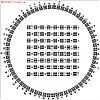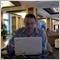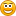# Elliot Wave Indicators1041

request Wave Indicator or Elliott Wave

--

Files:
wave.jpg  97 kb1041

Files:
dd.jpg  97 kb224

Anyone have Elliott Wave indicator for MT4.0

I just saw people using it .

Because I know that the ADVANCED GET 8.0 has this function.

MT4.0 is also supposed to.

Files:
eurusd_1h.jpg  72 kbModerator
123473

grunge:
I just saw people using it .

Because I know that the ADVANCED GET 8.0 has this function.

MT4.0 is also supposed to.

I am not sure that it is indicators you need (attached).

Files:
evwma.mq4  3 kb236

newdigital:
I am not sure that it is indicators you need (attached).

do u have elliot wave oscillator explantion. if u have it then please give me sir

regards,

k.n.v.224

newdigital:
I am not sure that it is indicators you need (attached).

It might be

,

anyway

thank you .328

Elliott Wave Indicator Set

I have started looking into trading the elliott wave however i would like an indicator set for MT4.

Here is some formulas that i found on another site:

I am hoping somebody could help me to add some Elliot Wave indicators to my MT studies.

1. Elliot Wave oscillator (EWO)

Parameters: hasn’t parameter.

Calculation: EWO (i) = MVA((high + low) / 2, 5) - MVA((high + low) / 2,

35).

2. Elliot Wave trend (EWTREND)

Parameters: N - number of periods, TR - trigger.

Calculation: EWTREND (i) = f (last(i), high(i), low(i), TR), where f is

logical expression,

high(i) = max (last(j)), low(i) = min (last(j)), j = ,

last(j) = MVA((high + low) / 2, 5) - MVA((high + low) / 2, 35).

3. Elliot Wave number (EWN)

Parameters: N - number of periods, TR - trigger.

Calculation: EWN (i) = f (last(i), high(i), low(i), TR), where f is

logical expression,

high(i) = max (last(j)), low(i) = min (last(j)), j = ,

last(j) = MVA((high + low) / 2, 5) - MVA((high + low) / 2, 35).

There are three indicator sets and the trading rules are as follows:

Long when:

1. EWN is a 'new' 3 or 5

2. EWTrend is a 0 or 1

3. EWO is positive

These 3 things must happen simultaneously

Short when:

1.EWN is a 'new' 4

2.EWTrend is a 0 or -1

3.EWO is negative

These 3 things must happen simultaneously

Exit when:

1.EWO goes from - to + or from + to -

This should work in any time frame : 15 mins seem to work very well, but try other ones e.g. 5 mins and 1 hr.

Thanx for any and all help,

Graham

Files:
elliott.gif  49 kbModerator
123473

I moved this thread to the "Suggestions for Trading Systems" area to find it easy.4413

EW TREND

inputs: period(numeric),trigger(numeric);

vars trend(0), osc(0);

osc = EWO

if osc = @highest(osc,period) and trend = 0 then trend = 1

if osc = @lowest(osc-period) and trend = 0 then trend = -1

if @lowest(osc,period) < 0 and trend = -1 and osc < and trend -1 and

osc > -1*trigger*lowest(osc,period) then trend = -1

vice versa for highest

EWTREND=trend;

this might help44

FXJJ:
EW TREND

inputs: period(numeric),trigger(numeric);

vars trend(0), osc(0);

osc = EWO

if osc = @highest(osc,period) and trend = 0 then trend = 1

if osc = @lowest(osc-period) and trend = 0 then trend = -1

if @lowest(osc,period) < 0 and trend = -1 and osc < and trend -1 and

osc > -1*trigger*lowest(osc,period) then trend = -1

vice versa for highest

EWTREND=trend;

this might help

Ok lets see if this helpsJack..